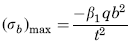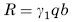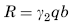Related Resources: calculators

### Loading Uniformly Decreasing to 1/3b Stress and Deflection Equation and Calculator.

Flat Rectangular Plate, Three Edges Fixed, One Edge (a) Free Loading Uniformly decreasing from fixed edge to zero at 1/3 b Stress and Reaction Loading Equation and Calculator.

Per. Roarks Formulas for Stress and Strain for flat plates with straight boundaries and constant thickness

 Rectangular plate; Three Edges Fixed One Edge FreeLoading Uniformly decreasing from fixed edge to zero at 1/3 bAt x = 0, z = 0
Stress and Reaction ForceReaction ForceStress and Reaction Force at x = 0, z = bReaction ForceWhere used:
E = Modulus of Elasticity (lbs/in2)
q = Total load or force to Plate (lbs/in2)
v = Poisson’s ratio (assumed to be 0.3)
t = plate thickness, (in)
a = plate length, (in)
b = plate width, (in)
σb = stress, (lbs/in2)
R = Reaction Force (lbs/in)
β1,2 = Constant From Table A
γ1,2 = Constant From Table A

Table A

 a/b 0.25 0.5 0.75 1 1.5 2 3 β1 0.014 0.035 0.047 0.061 0.075 0.08 0.082 β2 0.01 0.024 0.031 0.03 0.025 0.02 0.013 γ1 0.088 0.13 0.146 0.155 0.161 0.162 0.162 γ2 0.046 0.069 0.079 0.077 0.074 0.074 0.082

Reference:

Roarks Formulas for Stress and Strain, 7th Edition